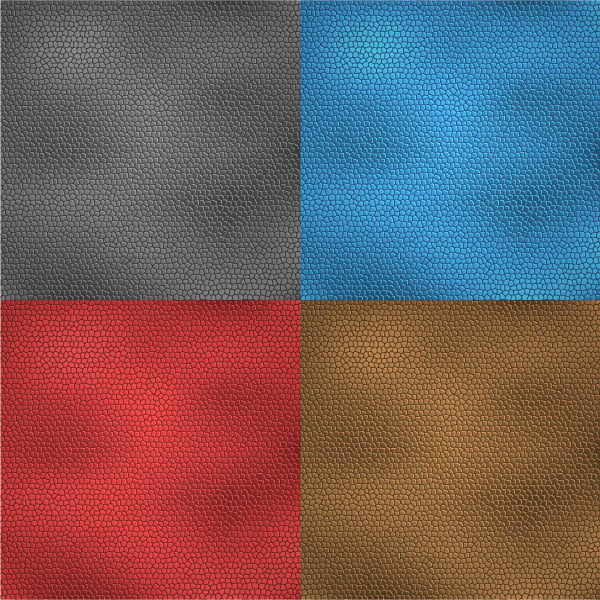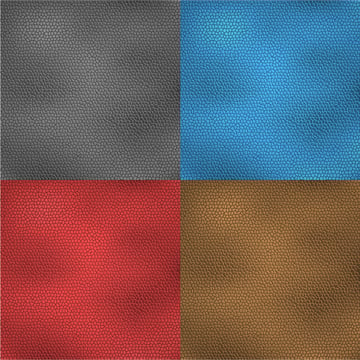In this tutorial I will show you how to create a leather texture of different colors using Adobe Illustrator. This is a basic tutorial to follow and will take around thirty minutes to complete. You can use this vector texture in your artwork when you need to add a leather texture. Let's get started.

## Step 1

Create a new document with File > New (Command + N).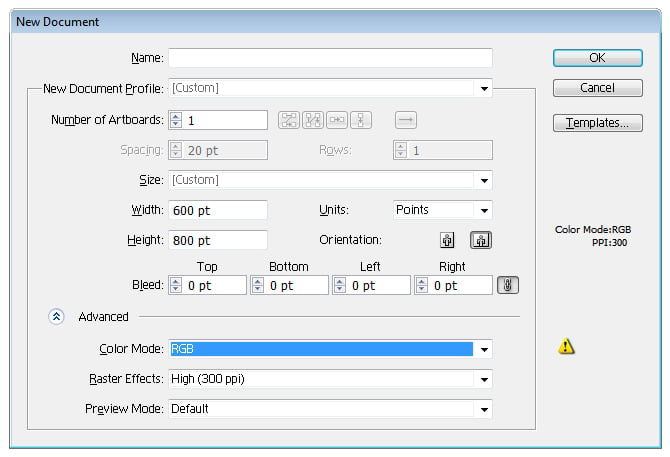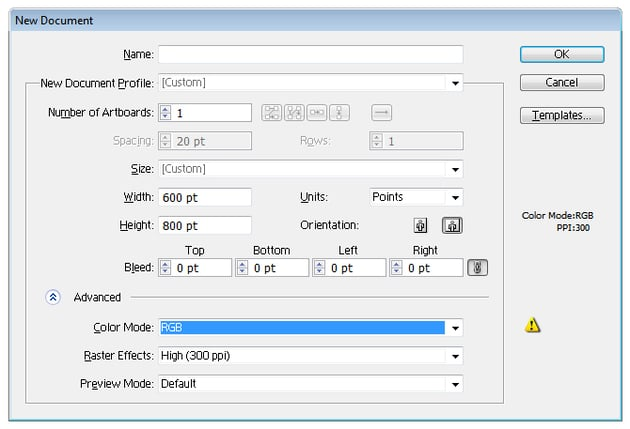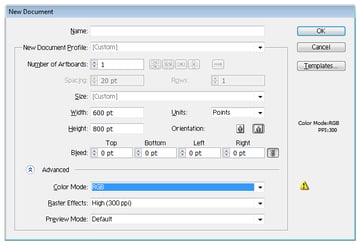## Step 2

Use the Rectangle Tool (M) to create a square with the settings shown below. Set the stroke to none, color it with RGB (R=255, B=255, G=255) and position it to center.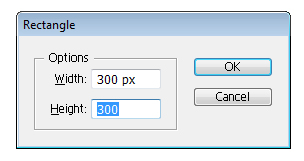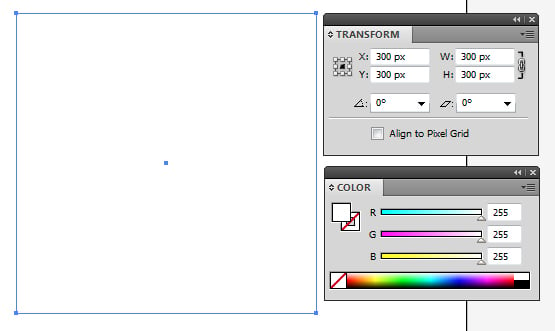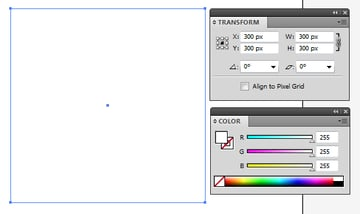## Step 3

Go to Effect > Texture > Stained Glass..., a window opens and then click OK to apply it. Go to Object > Expand Appearance.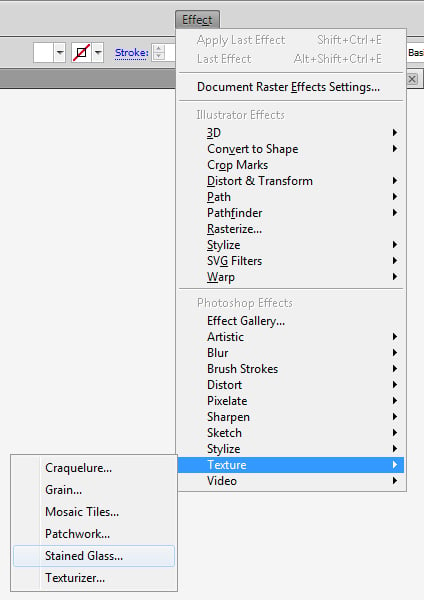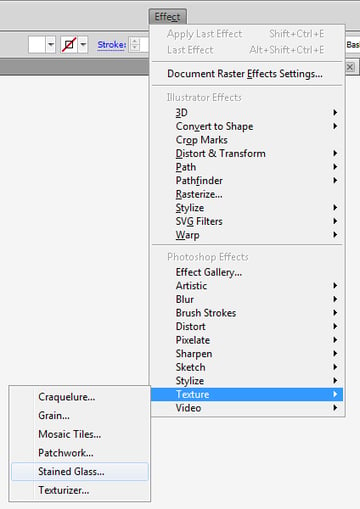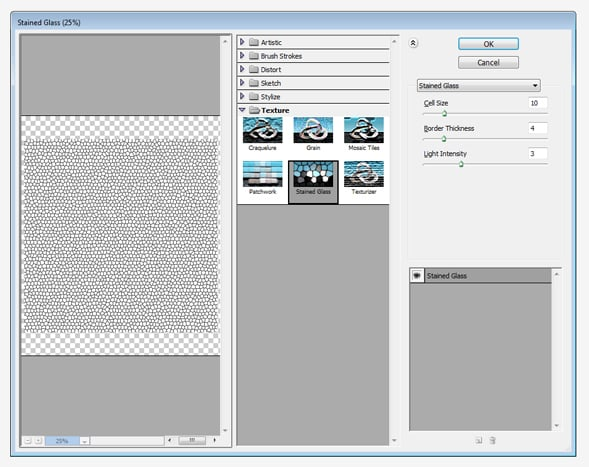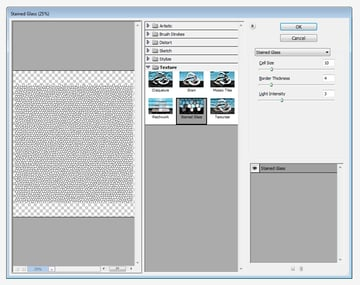## Step 4

Go to Object > Live Trace > Make and Expand.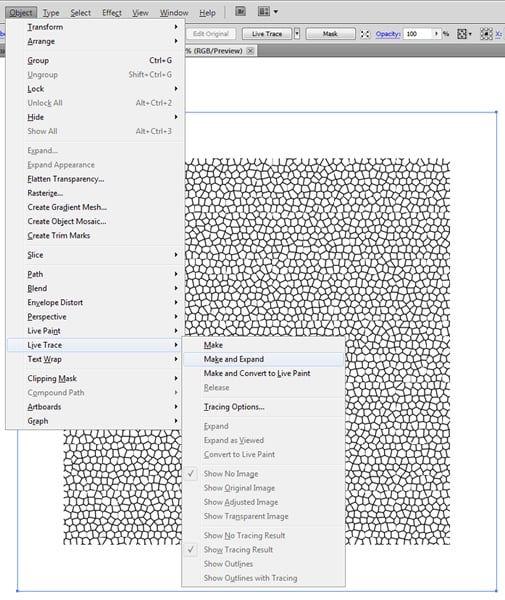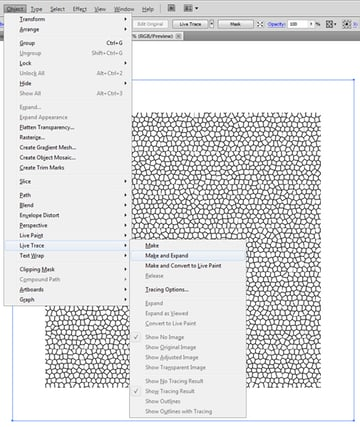## Step 5

Right-click and Ungroup the layer.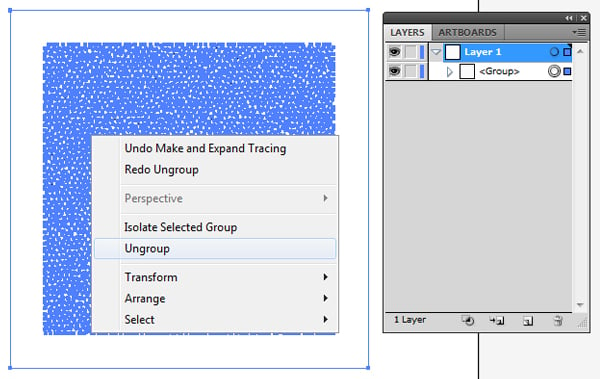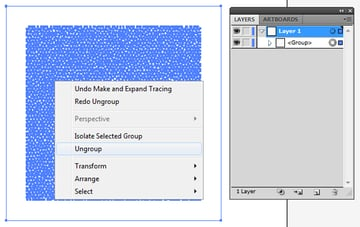## Step 6

Use Magic Wand Tools (Y), select the white colors and delete.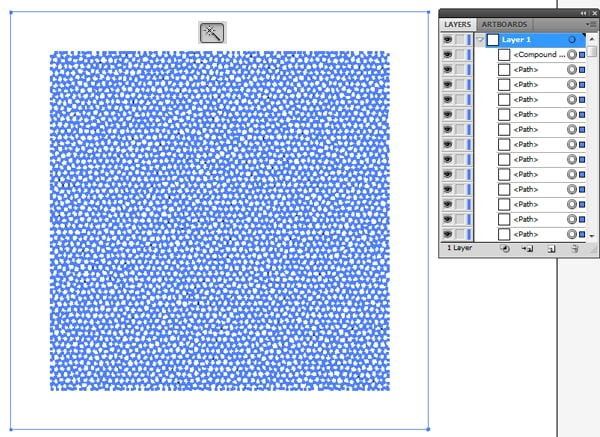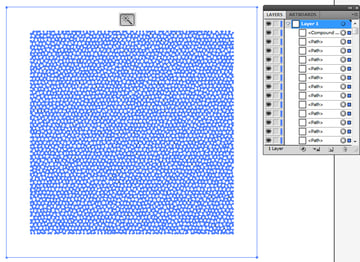## Step 7

Color the layer with RGB (R=25, G=25, B=25), position it in the center of artboard, and modify the size to 300 px. Uncheck the constrain proportion.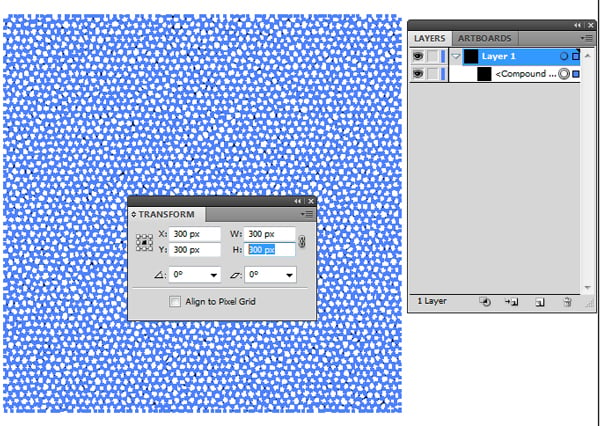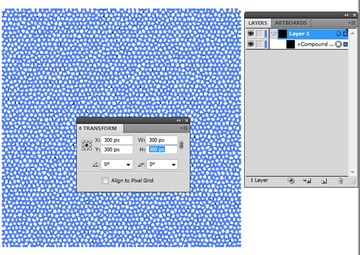## Step 8

Make a copy of the layer (Command + C) and paste in back (Command + B). Color the layer with RGB (R=65, G=65, B=65), and modify its position: transform x=301 and y=299.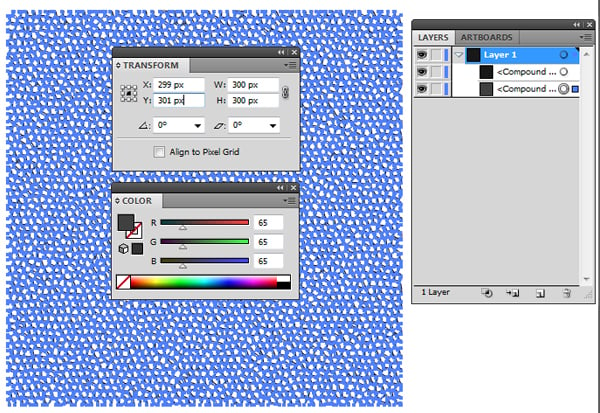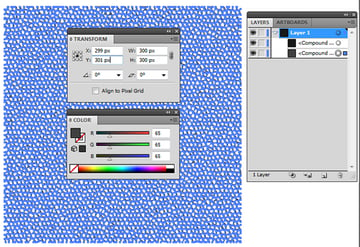## Step 9

Use the Rectangle Tool (M) to create a square with the following settings: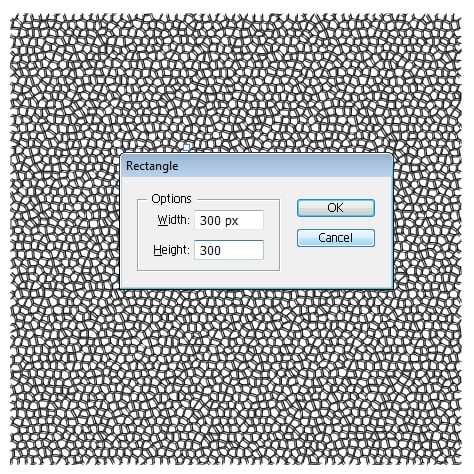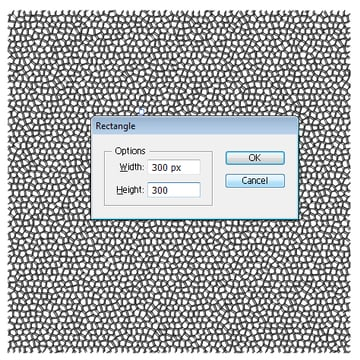## Step 10

Color the layer with RGB (R=40, B=40, G=40), and modify its position in the center of artboard as x=300 and y=300. Move the order layer down as shown.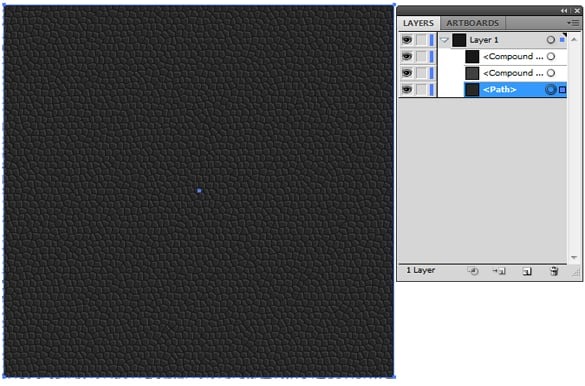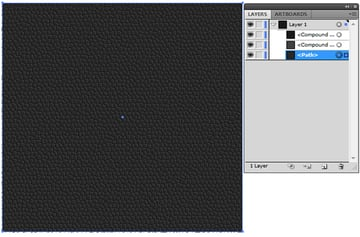## Step 11

Make a copy of the layer (Command + C) and Paste in Front (Command + F). Keep the Command key pressed to select the layers in the image below.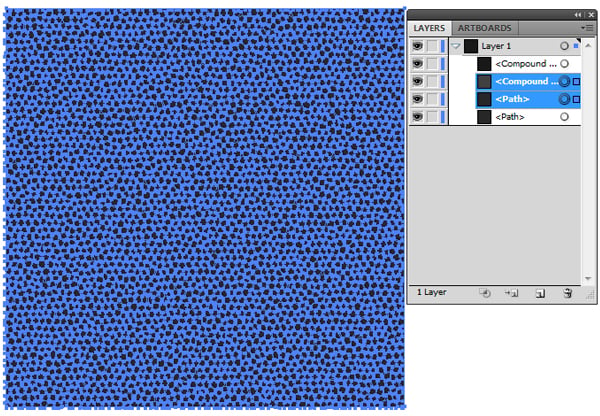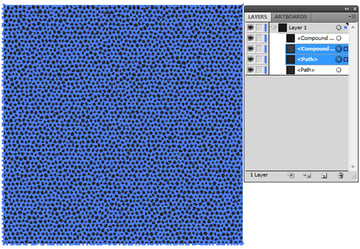## Step 12

Keep the Alt key pressed to select Intersect from the Pathfinder window and Expand.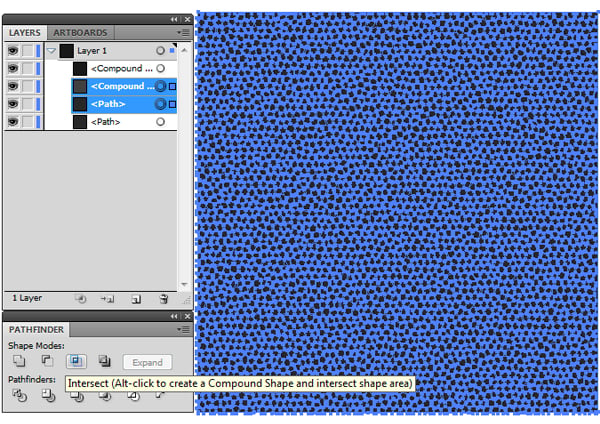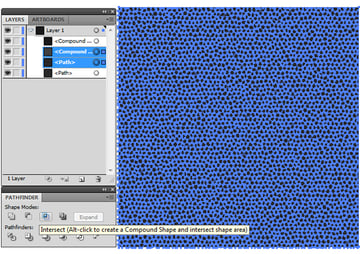## Step 13

Make a copy of the bottom layer (Command + C) and Paste in Front (Command + F). Select the layer you just copied and go to Object > Create Gradient Mesh. Enter the data shown in the following image and click OK.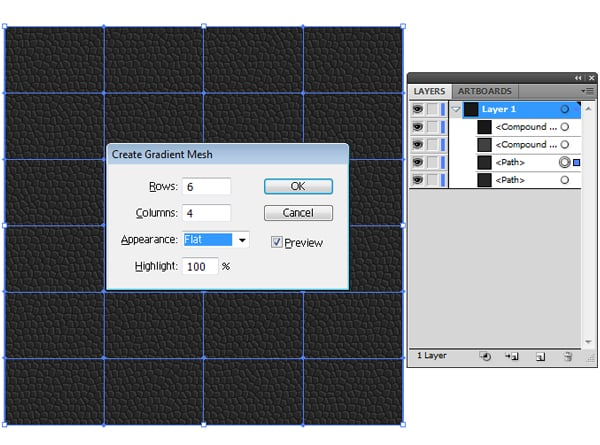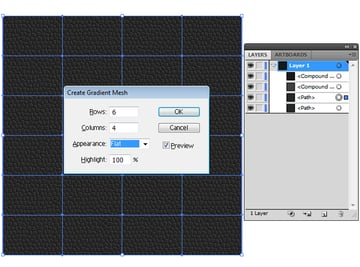## Step 14

Lock the top layers.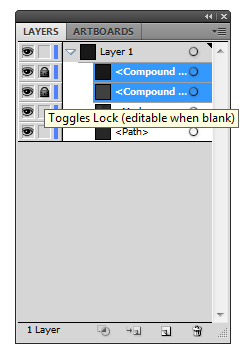Now color the mesh points with the following colors: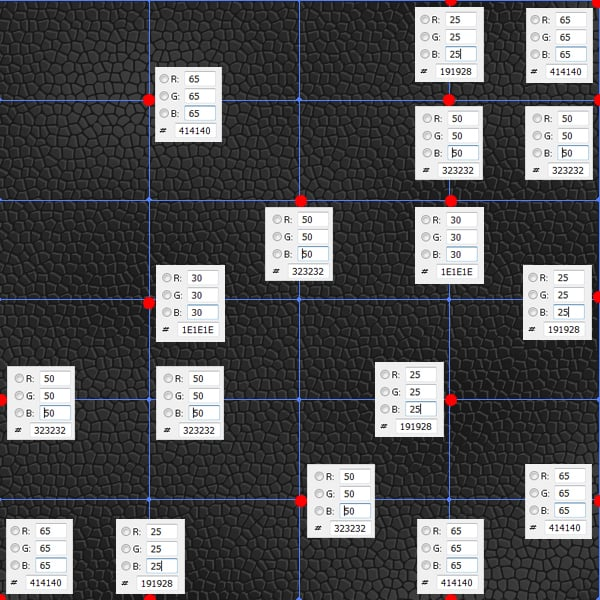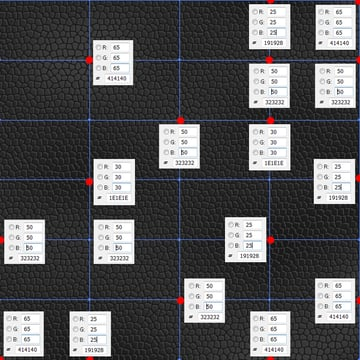## Step 15

Now after you have finished coloring you can unlock the layers. Select the Ellipse Tool and click on the artboard to create a circle with the following settings: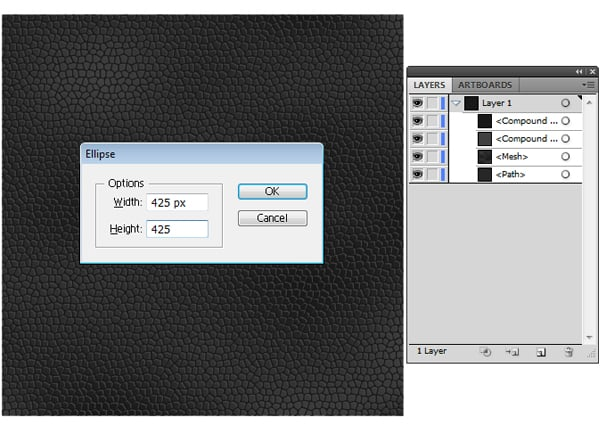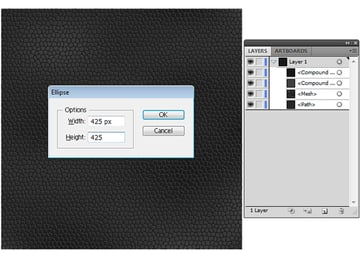## Step 16

Color the circle with the Gradient Tool (G), using the following colors: RGB (R=255, G=255, B=255) and RGB (R=150, G=150, B=150) with a type of Radial. Modify its position in the center of artboard x=300 and y=300, then select Overly from the Transparency window.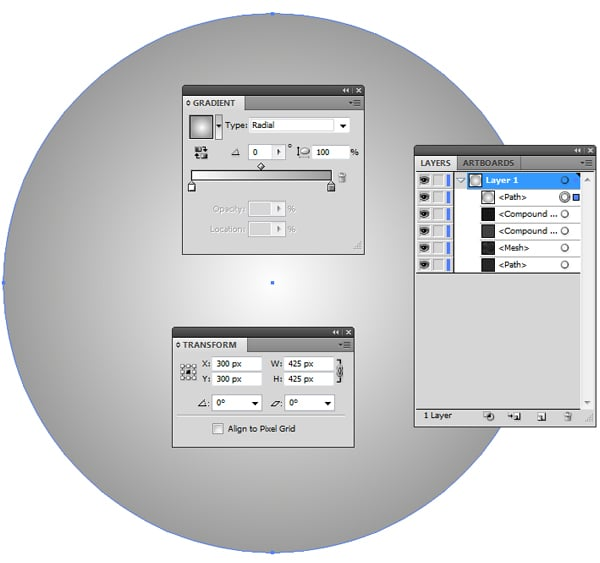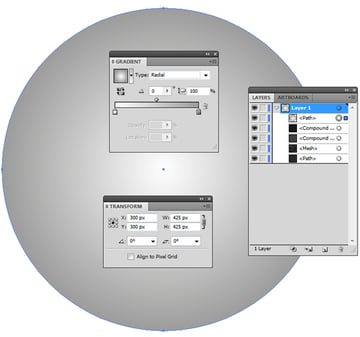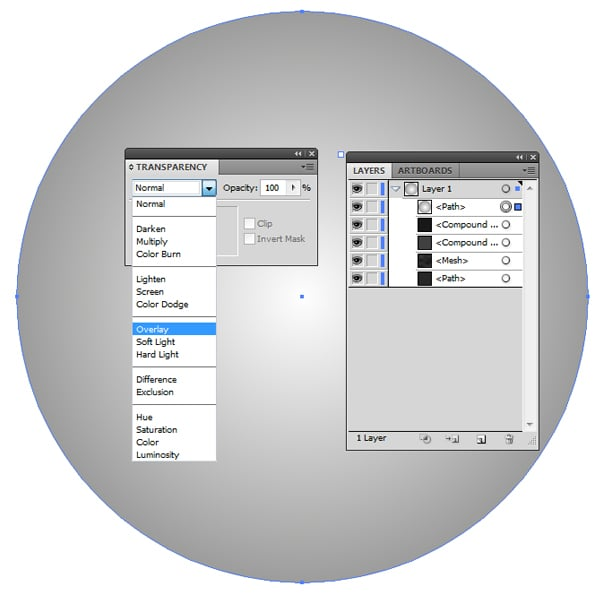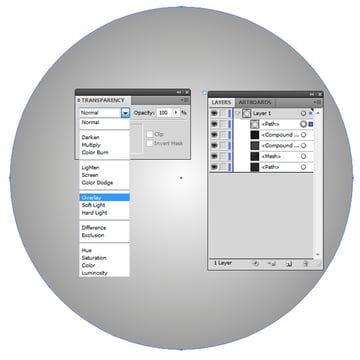## Step 17

Use the Rectangle Tool (M) to create a square with the following settings: x=300 and y=300, then modify its position in the center of the artboard: x=300 and y=300.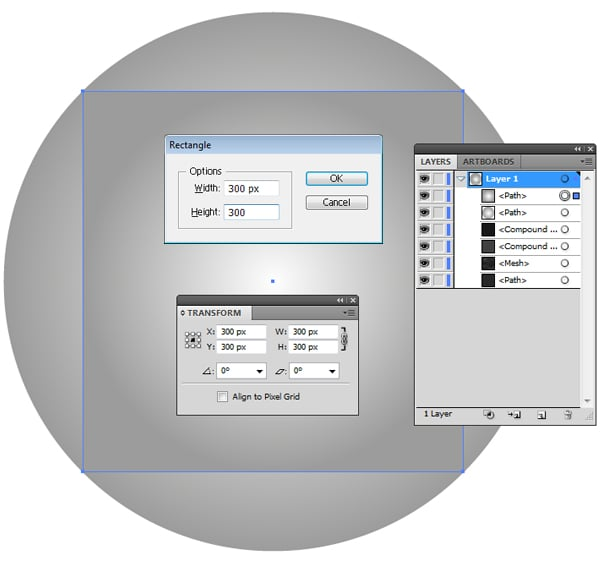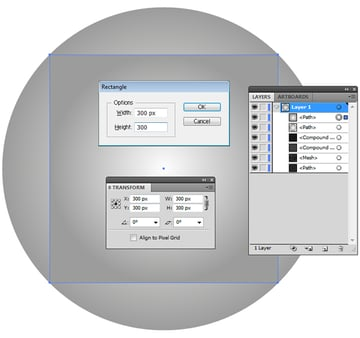## Step 18

Select the first two layers you just created and Right-click > Make Clipping Mask. Select all the layers and group them (Command + A > Command + G).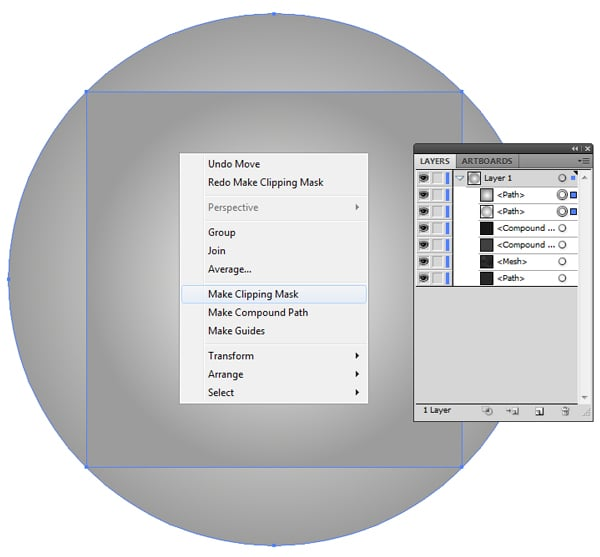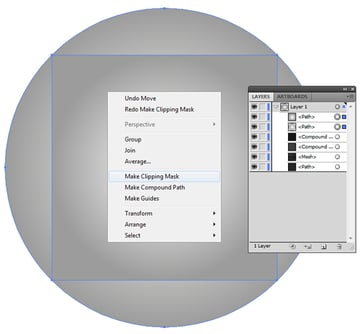## Step 19

Modify its position in the top right corner of artboard: x=150 and y=150.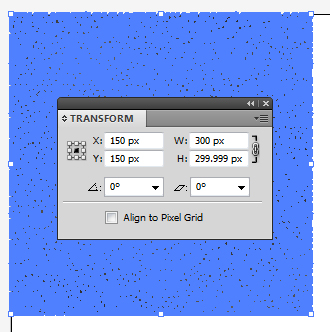## Step 20

Make a copy of the group layer (Command + C) and Paste in Front (Command + F). Modify its position in the top left corner of the artboard: x=450 and y=150.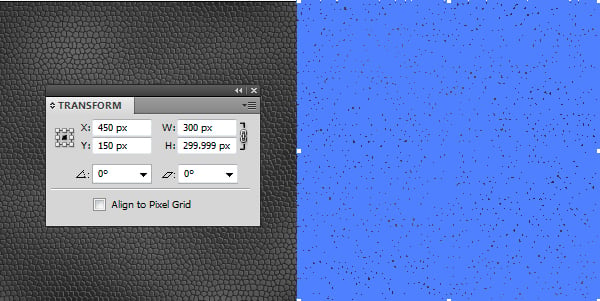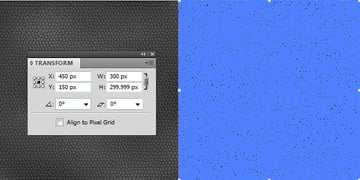## Step 21

Open the group and make a copy of the square for the bottom layer (Command + C) and Paste in Front (Command + F). Color the layer with RGB (R=75, G=175, B=255), select Overly in the Transparency window, and move up the layer to the second from the group, then close the group.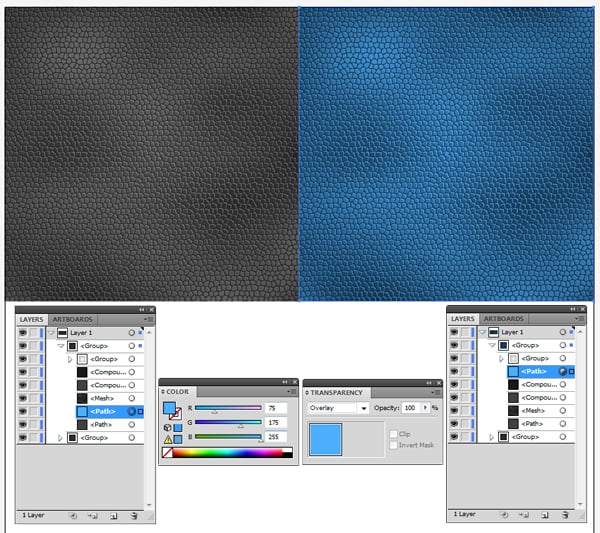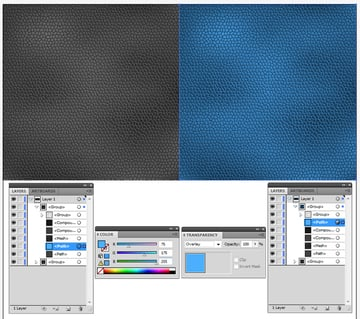## Step 22

Make a copy of the group layer (Command + C) and Paste in Front (Command + F). Modify its position in the bottom right corner of the artboard: x=150 and y=450. Change the RGB color (R=75, G=175, B=255) and layer of the group to a RGB color (R=255, G=75, B=75).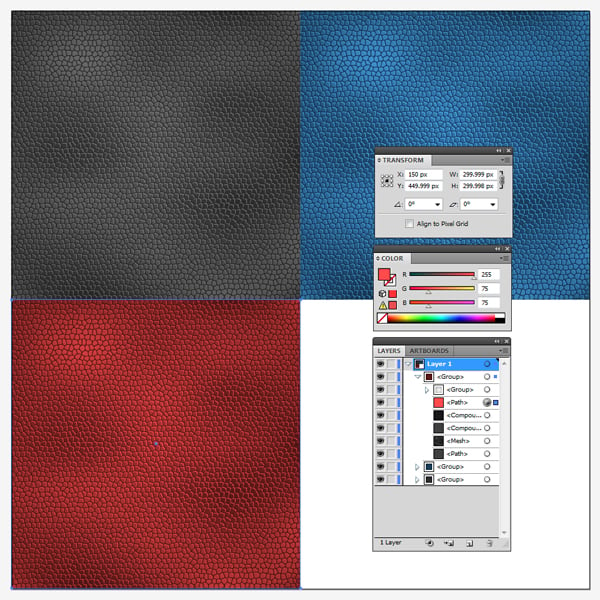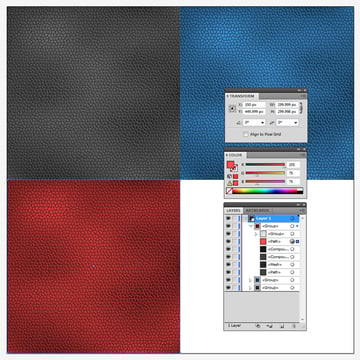## Step 23

Make a copy of the group layer (Command + C) and Paste in Front (Command + F). Modify its position in the bottom left corner of the artboard: x=450 and y=450. Change the RGB color (R=255, G=75, B=75) and layer of the group to RGB (R=175, G=125, B=75).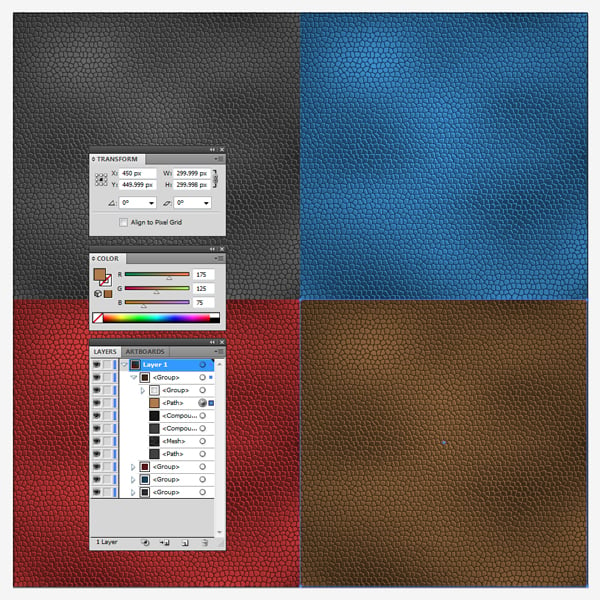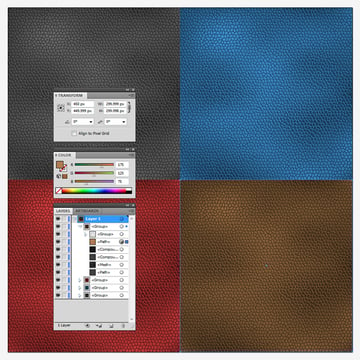## Conclusion

Now your leather texture with different colors is done. I hope you've enjoyed this tutorial.